Monday, 15 April 2013

Class 9 - Science - CH 8 - Motion (MCQs)

Motion

MCQs

Q1: A train moving with a uniform speed of 54 kmph. What is its speed in m/s?

(a) 15 m/s
(b) 1.5 m/s
(c) 9 m/s
(d) 90 m/s

Q2: A scalar quantity has ______________?

(a) magnitude only
(b) direction only
(c) both direction and magnitude
(d) none of these

Q3: When an object undergoes acceleration

(a) there is always an increase in its velocity
(b) there is always an increase in its speed
(c) a force always acting on it
(d) all of the above.

Q4: A truck covers 40 km with an average speed of 80km/h. Then it travels another 40 km with
an average speed of 40 km/h. The average speed of the truck for the total distanced covered is:

(a) 40 km/h
(b) 45km/h
(c) 48km/h
(d) 53km/h

Q5: The SI unit of retardation is

(a) ms-1
(b) ms-2
(c) ms2
(d) m

Q6: A car starts from city A and it travels 50 km in a straight line to city B. Immediately it turns around, and returns to city A. It took 2 hours for this round trip. The average speed of the car for this round trip is:

(a) 0 km/h
(b) 25 km/h
(c) 50 km/h
(d) 100 km/h

Q7: The equation v = u + at gives information as
(a) velocity is a function of time.
(b) velocity is a function of position.
(c) Position is a function of time.
(d) Position is function of velocity and time.

Q8: Which of the following can determine the acceleration of a moving object?

(a) area of velocity-time graph
(b) slope of velocity-time graph
(c) area of distance-time graph
(d) slope of the distance time-graph

Q9: A body is projected up with an initial velocity u m/s. It goes up to a height h metres in t seconds time. Then it comes back at the point of projection. Considering negligible air resistance, which of the following statement is true?

(a) the acceleration is zero
(b) the displacement is zero
(c) the average velocity is 2h/t
(d) the final velocity is 2u when body reaches projection point.

Q10: A car accelerates at 1.5 m/s2 in a straight road. How much is the increase in velocity in 4s?

(a) 6 m/s
(b) 4 m/s
(c) 3 m/s
(d) 2.66 m/s

1: (a) 15 m/s [Hint: 54×1000m/3600s = 15m/s]
2: (a) magnitude only
3: (c) a force always acting on it
4: (d) 53km/h
5: (b) ms-2
6: (c) 50 km/h
7: (a) velocity is a function of time.
8: (b) slope of velocity-time graph
9: (b) the displacement is zero
10:  (a) 6 m/s  [Hint use v - u = at]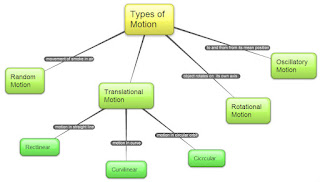1.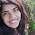nice questions

1.Thnks vaishnavi....we always appreciated ur effort.

2.Thnks vaishnavi....we always appreciated ur effort.

2.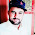3.1.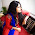This comment has been removed by the author.

4.5.These questions have helped me a lot

6.nice questions..............!! : )

7.It helps me a lot and also value based physics questions...thanks to who send it me

8.This article helps me a lot and it is also value based Ques.of physics.....thnks to whom help to send it
******

9.This article helps me a lot and it is also value based Ques.of physics.....thnks to whom help to send it
******

10.Very good it helped me a lot

11.i need download file for every chapter i need it for sa

12.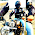Good questions...

13.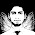Really good questions����
such questions so it'll be

14.Good questions but some what easier please give hard questions

15.Good questions but some what easier please give hard questions

16.Bakwaas don't give such stupid question waste of time

17.Bakwaas don't give such stupid question waste of time

18.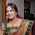This comment has been removed by the author.

19.Thanks for providing such a good questions , I scored 8 out of 10 marks in my class test 👍👍👍

20.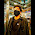really helped a lot

21.Helpful questions🖒. Now, I can give my exam very confidently😁